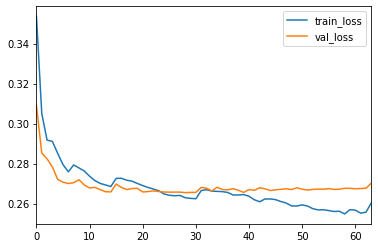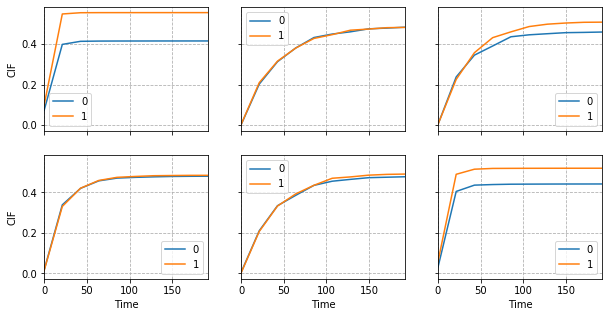# DeepHit with Competing Risks¶

In this notebook we give an example of how to apply the DeepHit method for competing risks.

The pycox package has (so far) limited support for competing-risk data, so the evaluation procedure at the end is somewhat limited.

In :
import numpy as np
import pandas as pd
import matplotlib.pyplot as plt
import torch
import torchtuples as tt

from pycox.preprocessing.label_transforms import LabTransDiscreteTime
from pycox.models import DeepHit
from pycox.evaluation import EvalSurv

In :
np.random.seed(1234)
_ = torch.manual_seed(1234)


## Dataset from the DeepHit repo¶

We download a competing risk data set from the DeepHit authors repo. The dataset is from a simulation study with two event types and censored observations.

We split in train, val and test.

In :
url = 'https://raw.githubusercontent.com/chl8856/DeepHit/master/sample%20data/SYNTHETIC/synthetic_comprisk.csv'
df_test = df_train.sample(frac=0.2)
df_train = df_train.drop(df_test.index)
df_val = df_train.sample(frac=0.2)
df_train = df_train.drop(df_val.index)

In :
df_train.head()

Out:
time label true_time true_label feature1 feature2 feature3 feature4 feature5 feature6 feature7 feature8 feature9 feature10 feature11 feature12
1 1 0 1 1 0.015579 -0.84608 0.48753 0.65193 0.20099 -0.11238 -1.39630 -0.188740 -0.30001 -0.24032 -0.38533 -1.02450
2 34 2 34 2 0.446490 1.64100 -1.74500 0.31795 -1.14060 0.36560 0.28110 -0.582530 -1.69070 1.20220 -0.51920 1.78400
3 9 0 9 2 0.629460 -0.61575 -0.32345 -0.90020 0.45360 -0.61992 2.16240 0.198750 -1.11960 -2.73210 -0.25673 -0.81836
5 11 2 11 2 0.487010 0.52086 1.99370 -0.94736 0.24371 1.06550 0.57686 0.019192 0.23212 0.48023 -0.73096 1.43960
6 37 0 40 2 -1.183700 -0.31602 -0.58640 -0.53890 -1.15830 1.04010 0.61938 -0.415420 -0.50700 -2.18300 0.97320 0.97753

## Feature transforms¶

The covariates are standardized, so we don't need to any preprocessing.

In :
get_x = lambda df: (df
.drop(columns=['time', 'label', 'true_time', 'true_label'])
.values.astype('float32'))

In :
x_train = get_x(df_train)
x_val = get_x(df_val)
x_test = get_x(df_test)


### Label transforms¶

Currently, the pycox package is mainly focused on single-event data, so there is no dedicated label transformer for competing risks. So, we make a simple one on our own, based on the the transform for the discrete methods LabTransDiscreteSurv.

The class returns durations (as integers) and event types (as integers) where 0 is reserved for censored observations.

We discretize the data to num_durations time points, which can be found with labtrans.cuts.

In :
class LabTransform(LabTransDiscreteTime):
def transform(self, durations, events):
durations, is_event = super().transform(durations, events > 0)
events[is_event == 0] = 0
return durations, events.astype('int64')

In :
num_durations = 10
labtrans = LabTransform(num_durations)
get_target = lambda df: (df['time'].values, df['label'].values)

In :
y_train = labtrans.fit_transform(*get_target(df_train))
y_val = labtrans.transform(*get_target(df_val))
durations_test, events_test = get_target(df_test)
val = (x_val, y_val)

In :
y_train[:6], y_train[:6]

Out:
(array([0, 2, 0, 1, 1, 1]), array([0, 2, 0, 2, 0, 1]))
In :
labtrans.cuts

Out:
array([  0.        ,  21.33333333,  42.66666667,  64.        ,
85.33333333, 106.66666667, 128.        , 149.33333333,
170.66666667, 192.        ])

## Network architecture¶

Under, we describe two networks that can be for competing risks:

The first, SimpleMLP, is a regular MLP which outputs a [batch_size x num_risks x num_durations], e.g., [64, 2, 10] tensor.

The other, CauseSpecificNet is similar to the cause-specific network described in the DeepHit paper. It has the same output shape as the SimpleMLP, but is a little more complex to make.

In :
class SimpleMLP(torch.nn.Module):
"""Simple network structure for competing risks.
"""
def __init__(self, in_features, num_nodes, num_risks, out_features, batch_norm=True,
dropout=None):
super().__init__()
self.num_risks = num_risks
self.mlp = tt.practical.MLPVanilla(
in_features, num_nodes, num_risks * out_features,
batch_norm, dropout,
)

def forward(self, input):
out = self.mlp(input)
return out.view(out.size(0), self.num_risks, -1)

In :
class CauseSpecificNet(torch.nn.Module):
"""Network structure similar to the DeepHit paper, but without the residual
connections (for simplicity).
"""
def __init__(self, in_features, num_nodes_shared, num_nodes_indiv, num_risks,
out_features, batch_norm=True, dropout=None):
super().__init__()
self.shared_net = tt.practical.MLPVanilla(
in_features, num_nodes_shared[:-1], num_nodes_shared[-1],
batch_norm, dropout,
)
self.risk_nets = torch.nn.ModuleList()
for _ in range(num_risks):
net = tt.practical.MLPVanilla(
num_nodes_shared[-1], num_nodes_indiv, out_features,
batch_norm, dropout,
)
self.risk_nets.append(net)

def forward(self, input):
out = self.shared_net(input)
out = [net(out) for net in self.risk_nets]
out = torch.stack(out, dim=1)
return out

In :
in_features = x_train.shape
num_nodes_shared = [64, 64]
num_nodes_indiv = 
num_risks = y_train.max()
out_features = len(labtrans.cuts)
batch_norm = True
dropout = 0.1

# net = SimpleMLP(in_features, num_nodes_shared, num_risks, out_features)
net = CauseSpecificNet(in_features, num_nodes_shared, num_nodes_indiv, num_risks,
out_features, batch_norm, dropout)


## Training¶

We fit the net with the AdamWR cyclic optimizer with initial learning rate of 0.01, decoupled_weight_decay of 0.01, initial cycle length of 1 epoch, and multiply the learning rate with 0.8 and cycle length with 2 at each new cycle.

The hyperparameters in the DeepHit loss-function, alpha and sigma, are set to 0.2 and 0.1, respectively. Note that alpha here controls the convex combination of the two losses, $$\text{loss} = \alpha \text{loss}_\text{NLL} + (1 - \alpha) \text{loss}_\text{rank},$$ and therefore has a different interpretation than in the DeepHit paper.

In :
optimizer = tt.optim.AdamWR(lr=0.01, decoupled_weight_decay=0.01,
cycle_eta_multiplier=0.8)
model = DeepHit(net, optimizer, alpha=0.2, sigma=0.1,
duration_index=labtrans.cuts)

In :
epochs = 512
batch_size = 256
callbacks = [tt.callbacks.EarlyStoppingCycle()]
verbose = False # set to True if you want printout

In :
%%time
log = model.fit(x_train, y_train, batch_size, epochs, callbacks, verbose, val_data=val)

CPU times: user 3min 53s, sys: 19.9 s, total: 4min 13s
Wall time: 2min 2s

In :
_ = log.plot()## Evaluation¶

There is, currently, limited support for competing risks in pycox, so the evaluation here is just illustrative and should not be considered best practice.

The survival function obtained with predict_surv_df is the probability of surviving any of the events, and does, therefore, not distinguish between the event types. This means that we evaluate this "single-event case" as before.

In :
surv = model.predict_surv_df(x_test)
ev = EvalSurv(surv, durations_test, events_test != 0, censor_surv='km')

In :
ev.concordance_td()

Out:
0.7202672608491353
In :
ev.integrated_brier_score(np.linspace(0, durations_test.max(), 100))

Out:
0.10413137528575585

## The cumulative incidence function¶

The cumulative incidence function, or CIF, is commonly used in settings with competing risks. We can evaluate the case-specific concordance using these CIF's.

We use 1 - cif here because the CIF increases with with risk (while the survival function decreases with higher risk).

In :
cif = model.predict_cif(x_test)
cif1 = pd.DataFrame(cif, model.duration_index)
cif2 = pd.DataFrame(cif, model.duration_index)

In :
ev1 = EvalSurv(1-cif1, durations_test, events_test == 1, censor_surv='km')
ev2 = EvalSurv(1-cif2, durations_test, events_test == 2, censor_surv='km')

In :
ev1.concordance_td()

Out:
0.7150254240668652
In :
ev2.concordance_td()

Out:
0.712722920851343

## Plot CIF¶

Finally, we plot six random individuals and their cumulative incidence functions

In :
sample = np.random.choice(len(durations_test), 6)
fig, axs = plt.subplots(2, 3, True, True, figsize=(10, 5))
for ax, idx in zip(axs.flat, sample):
pd.DataFrame(cif.transpose()[idx], index=labtrans.cuts).plot(ax=ax)
ax.set_ylabel('CIF')
ax.set_xlabel('Time')
ax.grid(linestyle='--')In [ ]: